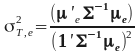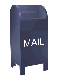### Global Minimum Variance Weights

Derivation of the global minimum variance equation for long/short portfolio weights. Here the only constraint is that the weights must sum to 1.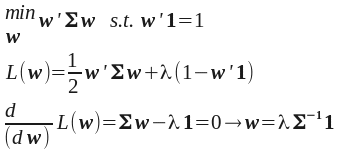multiplying both sizes by 1' (substituting into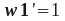)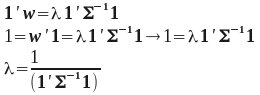substituting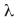into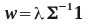we get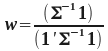### Tangency Portfolio

Weights for T, the tangency portfolio (where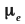) is the portfolio excess return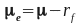: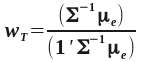Mean return of T: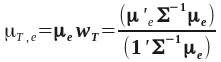Volatility of T: